# Multiplication + subtracting - math problems

#### Number of problems found: 53

• Expression 1What is 7+8-(5×2)+5-4+(6×(5-3)+6)-(8+10)-7+6?
• Evaluate expressionIf x=2, y=-5 and z=3 what is the value of x-2y
• How manzBy how many is the product of the numbers 328 and 7 greater than its sum?
• Brackets 2Add parenthesis to make true: 5-2×6-4+2=5
• Numbers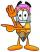By how many is the difference of numbers 8 and 34 less than its product?
• Operations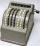Sum of the numbers 1.01 and 3.35 multiply by the difference of numbers 6.69 and 1.39.
• Expression 8Evaluate this expressions: a) 5[3 + 4(2.8 - 3)] b) 5×(8-4)÷4-2How much and how many times is 72.1 greater than 0.00721?
• Expression 6Evaluate expression: -6-2(4-8)-9
• EricaErica bought 3 1/2 yards of fabric. If she uses 2/3 of the fabric, how much will she have left?
• EmployeesOf all 360 employees, there are 11/12 women. How many men work in a company?
• Mixing operations with numbersSubtract twice the number -23.6 from the difference of the numbers -130 and -40.2.
• With bracket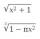Calculate (evaluate) simple mathematical expression with a negative numbers and a bracket: 13+15*5-2*(-6)
• In the bowlThere are 12 pears in the bowl. Erik ate a third. How many pears remained in the bowl?
• Boys and girls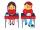In the class are 20 boys and 5-times less girls. How many girls are in the classroom? How many all children are in the class?
• Algebra problemThis is algebra. Let n represent an unknown number. 1. Eight more than the number n 2. Three times the number n 3. The product of the number n and eight 4. Three less than the number n 5. Three decreased by the number n
• Product of the sum and differenceCalculate the product of the sum and difference of numbers -7 and -2.
• Saving moneyMarko saved € 64. Milan saved 8 euros less than Marko. Marika saved 6 times more than Milan. Calculate who saved how much.
• RootThe root of the equation ? is (equal or greater or less than zero)? ?
• Grandma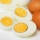Grandma bought two 10 pack of eggs. She used 8 eggs on the cake, 2 times less on the omelette and 4 more eggs for breakfast. How many eggs did it stand for?

Do you have an interesting mathematical word problem that you can't solve it? Submit a math problem, and we can try to solve it.

We will send a solution to your e-mail address. Solved examples are also published here. Please enter the e-mail correctly and check whether you don't have a full mailbox.

Please do not submit problems from current active competitions such as Mathematical Olympiad, correspondence seminars etc...

Multiplication Problems. Subtracting Problems.